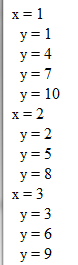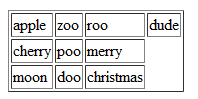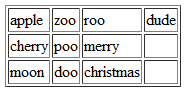In using this code "How do I display query results in an N-column table layout?" for the coldfusioncookbook site, I was wondering how one could modify it so that it also uses up a specific number of rows as well.

Meaning I want all of column one (10 rows) filled in first before creating column two and so on. Any ideas?

Right now I have only 6 records to display and they are using up 3 columns and two rows, I would want 1 column and 6 rows and then when it hits say 10, then start next column. Hope that's clear. That's for the great work!!

This was one of those weird questions that seemed so simple but took a while for me to wrap my brain around it. I decided to draw out a solution first:If you can actually read my horrible writing there, you can see I've got a table where each column is 3 cells high. I counted down 10 array elements and moved over one column when I got to the end. Looking at this it occured to me that I was probably looping over my array and incrementing by the size of the column. With that in mind, I first wrote a quick test.

`<cfscript> data = ["apple","cherry","moon","zoo","poo","doo","roo","merry","christmas","dude"]; colSize=3;`

`//First for(x=1; x<=colSize; x++) { writeOutput("x = #x#<br>"); for(y=x; y<=arrayLen(data); y+=colsize) { writeOutput("  y = #y#<br>"); } } </cfscript>`

My outer loop goes from one to colSize whereas the inner loop will loop over the array of data. It should "jump" like my hand written example did. Here's what I got:It looked ok to me, so I then worked up this version that made a table.

`//Second result = "<table border=""1"">"; for(x=1; x<=colSize; x++) { result &= "<tr>"; for(y=x; y<=arrayLen(data); y+=colsize) { result &= "<td>#data[y]#</td>"; } result &= "</tr>"; } result &= "</table>"; writeOutput(result);`

This version pretty much follows the last version, but now generates a table instead. It creates this:Woot. But now those empty table cells are kind of bugging me. Let's try this one more time...

`//Third result = "<table border=""1"">"; for(x=1; x<=min(arrayLen(data),colSize); x++) { result &= "<tr>"; thisPad = 0; for(y=x; y<=arrayLen(data); y+=colsize) { result &= "<td>#data[y]#</td>"; thisPad++; } if(x==1) { pad = thisPad; } else { if(pad > thisPad) result &= repeatString("<td> </td>", pad-thisPad); } result &= "</tr>"; } result &= "</table>"; writeOutput(result);`

The main difference now is a pad and thisPad variable. Pad is used to remember the width of the table and thisPad the size of each individual row. If I'm working on row 2-N I simply have to pad the string with empty cells. And the result...I don't know if this code will ever actually be useful, but it was fun to write. Here's the entire template.

`<cfscript> data = ["apple","cherry","moon","zoo","poo","doo","roo","merry","christmas","dude"]; colSize=3;`

`//First for(x=1; x<=colSize; x++) { writeOutput("x = #x#<br>"); for(y=x; y<=arrayLen(data); y+=colsize) { writeOutput("  y = #y#<br>"); } }`

`writeoutput("<p/><hr/><p/>");`

`//Second result = "<table border=""1"">"; for(x=1; x<=colSize; x++) { result &= "<tr>"; for(y=x; y<=arrayLen(data); y+=colsize) { result &= "<td>#data[y]#</td>"; } result &= "</tr>"; } result &= "</table>"; writeOutput(result);`

`writeoutput("<p/><hr/><p/>");`

`//Third result = "<table border=""1"">"; for(x=1; x<=min(arrayLen(data),colSize); x++) { result &= "<tr>"; thisPad = 0; for(y=x; y<=arrayLen(data); y+=colsize) { result &= "<td>#data[y]#</td>"; thisPad++; } if(x==1) { pad = thisPad; } else { if(pad > thisPad) result &= repeatString("<td> </td>", pad-thisPad); } result &= "</tr>"; } result &= "</table>"; writeOutput(result);`

`</cfscript>`